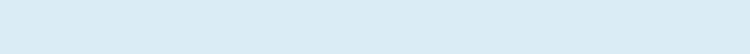402 year Cycle = 27 X 777 Sabbath = Solar & Sabbatical Sync

1 Sabbatical Year:

1 Week = 6 working days + 1 Sabbath day of rest = 7 Days

1 Month = 4 Weeks = 24 days + 4 Sabbaths = 28 Days

1 Year = 13 Months = 312 days + 52 Sabbaths = 364 Days

1  Solar Year = 365.2425 Days    Error = 1.2425 Days7 Sabbatical Year:

7 Years = 364 Days X 7 Years = 2,548 Days

7  Solar Years = 365.2425 X 7 = 2,556.6975 Days    Error = 8.6975 Days

7 Sabbatical Year = 2,548 + 7  = 2,555 Days: Final Error = 1.6975 Days28 Sabbatical Year:

4 X 7 Sabbatical Years = 4 X 2,555 Days = 10,220 Days

28  Solar Years = 365.2425 X 28 = 10,226.79 Days    Error = 6.79 Days

28 Sabbatical Years = 10,220 + 7 = 10,227 Days: Final error = -0.21 DayJubilee Year = 50 Sabbatical Year = 28 Sabbatical Year + 21 Sabbatical Year + 1 Year:

28 Sabbatical Years = 10,220 + 7 = 10,227 Days

21 Sabbatical Years = 3 X 7 Sabbatical Years = 3 X 2,555 = 7,665 Days

50 Years = 10,227 + 7,665 + 364 = 18,256 Days

50  Solar Years = 365.2425 X 49 = 18,262.125 Days    Error = 6.125 Days

50 Sabbatical Years = 18,256 + 7 = 18,263 Days: Final error = -0.875 DayGrand Jubilee Year = 8 X Jubilee Year

400 Years = 18,263 x 8 = 146,104 Days

400  Solar Years = 365.2425 X 400 = 146,097 Days    Error = -7 Days

400 Sabbatical Years = 146,104 - 7 = 146,097 Days Final error = 0 DayIf you divide 146,097 Days with 7, you will get 20,871 Sabbaths. If we divide 20,871 Sabbaths with 777,

you will get 26.8610039. If you multiply 27 times 777 times 7, that would be 146,853 Days.

So, 27 X 777 X Sabbath = 400 Sabbatical Years + 2 Sabbatical Years + 1 Sabbatical Month

That would be 402 Sabbatical Years + 1 Sabbatical Month

So, every 402 Years, the cycle will move by one month to another month.

See the 402 year cycle on the next page.